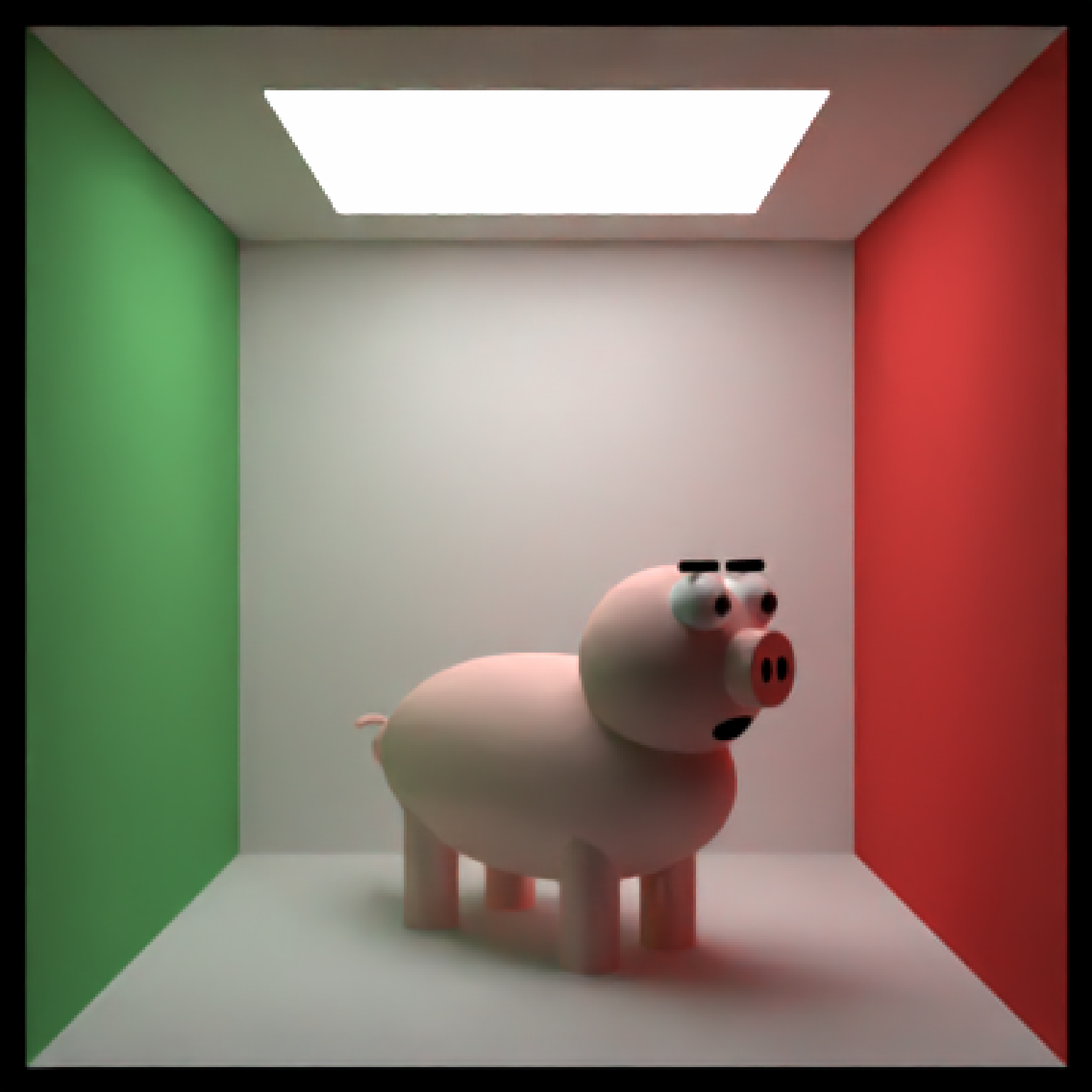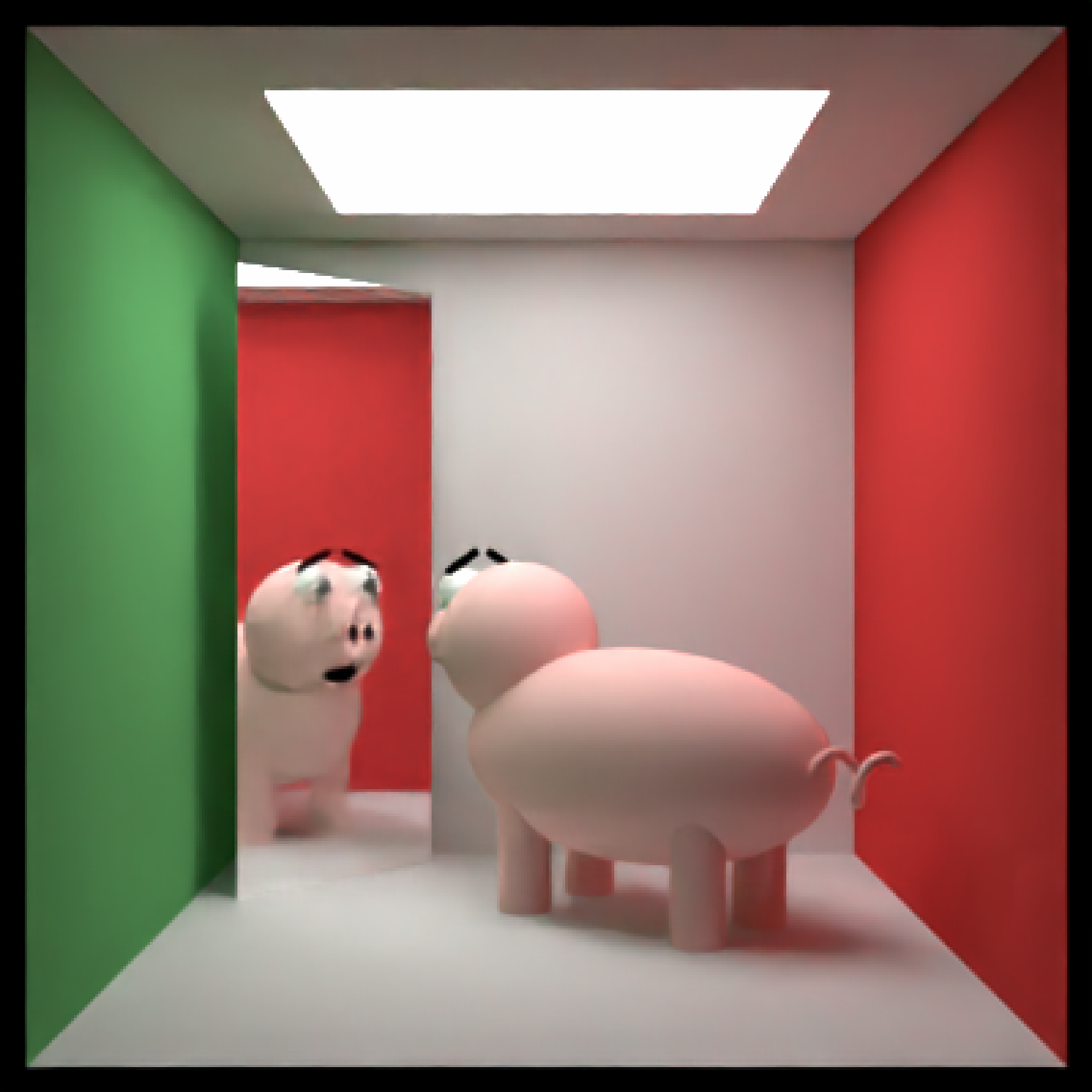Pig Object

pig(x = 0, y = 0, z = 0, emotion = "neutral", angle = c(0, 0, 0),
order_rotation = c(1, 2, 3), scale = c(1, 1, 1), diffuse_sigma = 0)

## Arguments

x Default 0. x-coordinate of the center of the pig. Default 0. y-coordinate of the center of the pig. Default 0. z-coordinate of the center of the pig. Default neutral. Other options include skeptical, worried, and angry. Default c(0, 0, 0). Angle of rotation around the x, y, and z axes, applied in the order specified in order_rotation. Default c(1, 2, 3). The order to apply the rotations, referring to "x", "y", and "z". Default c(1, 1, 1). Scale transformation in the x, y, and z directions. If this is a single value, number, the object will be scaled uniformly. Default 0. Controls the Oren-Nayar sigma parameter for the pig's diffuse material.

## Value

Single row of a tibble describing the pig in the scene.

## Examples

#Generate a pig in the cornell box.

generate_cornell() %>%
render_scene(parallel=TRUE, samples=400,clamp_value=10)#> Setting default values for Cornell box: lookfrom c(278,278,-800) lookat c(278,278,0) fov 40 .# Show the pig staring into a mirror, worried
generate_cornell() %>%
angle = c(0,-40,0), emotion = "worried")) %>%
angle = c(0,45,0), material = metal())) %>%
render_scene(parallel=TRUE, samples=500,clamp_value=10)#> Setting default values for Cornell box: lookfrom c(278,278,-800) lookat c(278,278,0) fov 40 .# Render many small pigs facing random directions, with an evil pig overlord
set.seed(1)
lots_of_pigs = list()

for(i in 1:10) {
lots_of_pigs[[i]] = pig(x=50 + 450 * runif(1), z = 50 + 450 * runif(1), y=50,
scale = c(30,30,30), angle = c(0,360*runif(1),0), emotion = "worried")
}

many_pigs_scene = do.call(rbind, lots_of_pigs) %>%
scale=c(100,100,100),angle=c(30,90,0), order_rotation=c(2,1,3)))render_scene(many_pigs_scene,parallel=TRUE,clamp_value=10, samples=500, tonemap = "reinhold")#> Setting default values for Cornell box: lookfrom c(278,278,-800) lookat c(278,278,0) fov 40 .